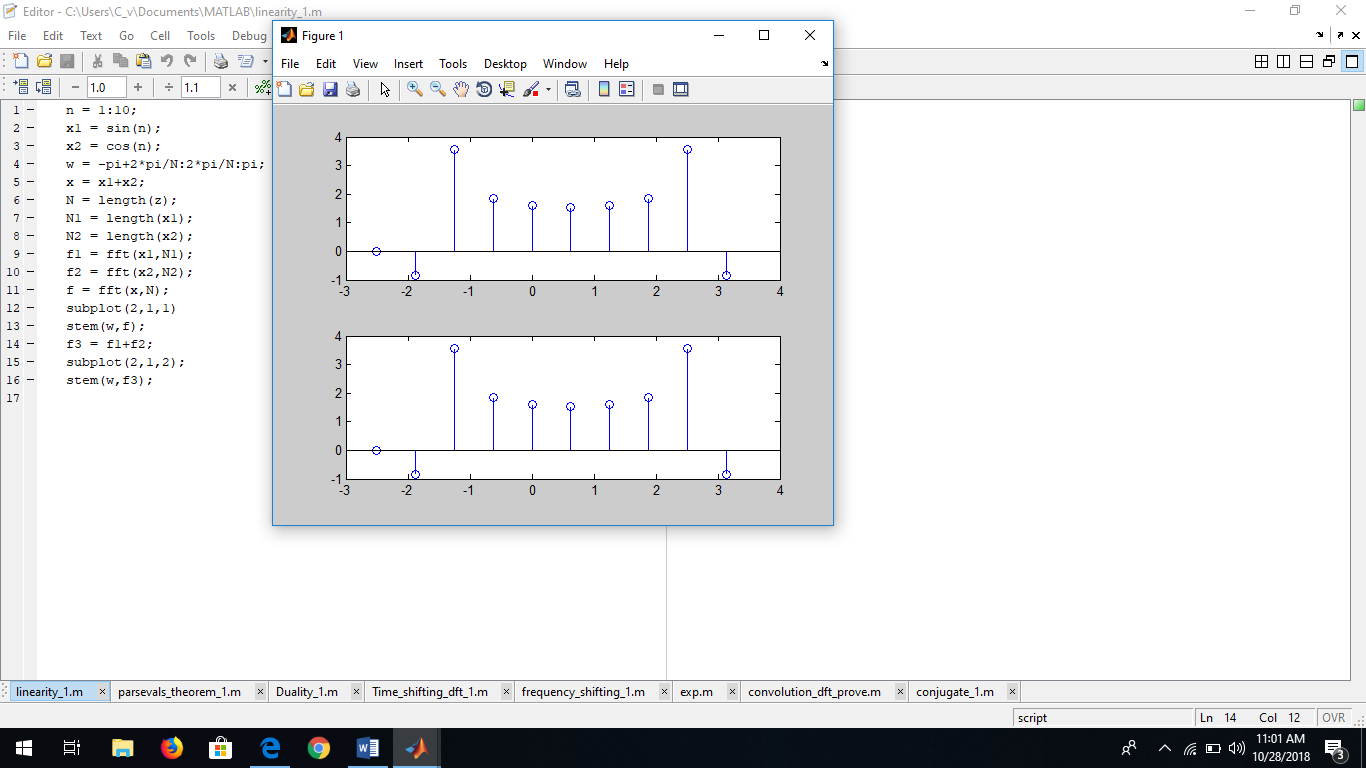# Linearity Property in MATLAB Complete Prove (Code + Output)

Linearity Property Prove: DFT{αx1(n) + βx2(n)} = αX1[K] + βX2[K]

You can prove Linearity Property in MATLAB of any sinusoidal signal. To do Linearity with any signal in Matlab, simply open and create a new Script (.m file) in Matlab.

Step 1:

Open MATLAB << Then on the top left corner click on the File option << Click New << Then click Script.

Step 2:

Now write the following Matlab code given below of Linearity Property into the new script (.m file) and save the file in the Matlab directory with any name you want.

LINEARITY PROPERTY MATLAB CODE:

n = 1:10;

x1 = sin(n);

x2 = cos(n);

w = -pi+2*pi/N:2*pi/N:pi;

x = x1+x2;

N = length(z);

N1 = length(x1);

N2 = length(x2);

f1 = fft(x1,N1);

f2 = fft(x2,N2);

f = fft(x,N);

subplot(2,1,1)

stem(w,f);

f3 = f1+f2;

subplot(2,1,2);

stem(w,f3);

Step 3:

Now Run the code, after you have saved the file.

OUTPUT:

energy_left =

5.0014

energy_right =

5.0014

FIGURE:This is how you can perform Prove Linearity Property in MATLAB of any signal. Hope you like this article. If you want to learn more about MATLAB you can read and view all the blog posts related to MALTAB here. MATLAB Learning

If you need any help and assistance, please comment down below so that we can help you. Good Luck!!

See More…